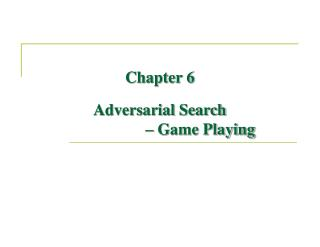DownloadDownload PresentationChapter 6 Adversarial Search – Game Playing

Chapter 6 Adversarial Search – Game Playing

Télécharger la présentationChapter 6 Adversarial Search – Game Playing

- - - - - - - - - - - - - - - - - - - - - - - - - - - E N D - - - - - - - - - - - - - - - - - - - - - - - - - - -
Presentation Transcript

1. Outline • Games of perfect information - perfect play • The minimax strategy • Multiplayer games • Alpha-Beta pruning • Games of imperfect information

2. Games • Competitive environments • goals of two agents are in conflict– adversarial search • Perfect play • deterministic and fully observable • turn-taking: actions of two players (agents) alternate • zero-sum: the utility values at the end of the game are equal and opposite (adversarial) • e.g., chess, winner (+1) and loser (-1) • Types of games

3. Define game as a search problem • initial state • the board position, the player to move, etc. • successor function • generates a list of (move, state) pairs • terminal test • decides when the game is over • terminal states: states when the game has ended. • utility function • gives a numeric value for the terminal states. • zero-sum games • game tree • defined by the initial state and the legal moves for each side

4. Game tree for the game of tic-tac-toe • High values are good for MAX and bad for MIN

5. Optimal contingent strategy • Optimal strategy • leads to outcomes at least as good as any other strategy when one is playing a infallible opponent – infeasible in practice. • 2-ply game • the tree is one move deep, consisting of two half-moves, each of which is a ply. • MAX’s moves in the states resulting from every possible response by MIN • minimax value of a node: the utility of being the corresponding state • MAX (MIN) prefers to move to a state of maximum (minimum) value. • minimax decision at the root.

6. The minimax algorithm • computes the minimax decision from the current state • recursion proceeds down to the leaves • minimax values are backed up

7. The property of the minimax algorithm • Complete? • Optimal? • Time? • Space?

8. Optimal decisions in multiplayer games • vector form: e.g. utility is <vA = 1, vB = 2, vC = 6> • pick up move (successor) having the highest value

9. Alpha-Beta Pruning • compute the minimax decision without looking at every node • pruning away branches that cannot possibly influence the final decision • Alpha: value of best choice for MAX • Beta: value of best choice for MIN

10. Alpha-Beta Pruning (cont’d)

11. Alpha-Beta Pruning (cont’d) • MINIMAX-VALUE (root) = max(min(3,12,8), min(2, x, y), min(14,5,2)) = max(3, min(2,x,y), 2) = max (3, z, 2) where z 2 = 3 • the value of the root (minimax decision) is independent of the values of the pruned leaves x and y. • depends on the order in which the successors are examined

12. How good is the Alpha-Beta pruning? e.g., try captures first, then threats, then forward moves, and then backward moves effective branching factor becomes

13. Imperfect decisions • Moves must be made in a reasonable (minutes) amount of time • Using Alpha-Beta pruning, the depth is still not practical if we insist on reaching the terminal states • should cut off the search earlier by applying a heuristicevaluation function to states • evaluation function estimates the utility of the position • use cut off test instead of terminal test • turning nonterminal nodes into terminal leaves

14. How to design good evaluation functions? • Requirements • order the terminal states in the same way as the true utility function • must not take too long • chances of winning • uncertain about the final outcomes because of the cut off • categories or equivalence classes of states: • the states have the same values, leading to wins, losses, or draws • the value of evaluation function should reflect the proportion of states with each outcome: wins (72%), losses (20%), or draws (8%) • weighted average (expected) value • requires experience and too many categories

15. How to design good evaluation functions? • In practice • computes separate numerical contributions from each feature and then combines them to find the total value • material value for each piece, e.g., pawn 1, knight/bishop 3, rook 5, queen 9 • weighted linear function • nonlinear combinations of features if the contribution of each feature is depends on values of the other features.

16. Deterministic games in practice